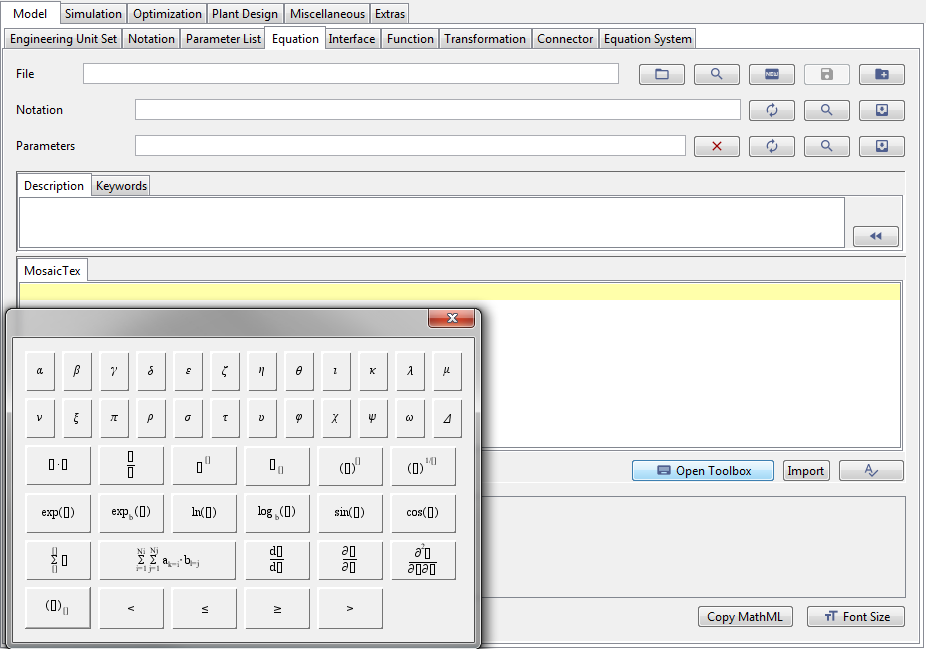# Model: Equation

Once you have defined base names etc. that appear in your equations, you can start to write the actual equations. Basic MOSAICmodeling models consist of equations with a single notation. These are then joined together to form an equation system.

### Purpose of the equation

This tab is used to actually write down the individual equations that you need in your model. In MOSAICmodeling, you formulate your equations in a LaTeX-based syntax. This means that you can actually see the mathematical expressions as an easily readable output during the formulation. The editor to create or edit an equation inside MOSAICmodeling is shown in Figure 1. This editor has a toolbox, which can be opened via the “Open Toolbox” button and can assist you in formulating equations.Figure 1: The equation editor inside MOSAICmodeling lets you formulate your equation and directly gives you a readable output of the current equation. Note that this editor might look differently depending on your operating system.Table 1: Overview of commands available in the toolbox

### Explanation of the equation editor

Table 2 explains the entries of the equation editor.

### Learning video

The learning video on equations is not yet available.